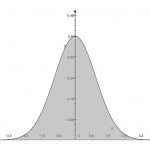# Analytic Implied Basis Point VolatilityBasis point volatility, or simply ‘BP Vol’, refers to the volatility parameter of the Normal, or Bachelier, model, $$dF=\sigma dW$$ I was interested to implement the implied volatility calculation using the analytic approximation of J. Choi, K Kim and M. Kwak (2009), “Numerical Approximation of the Implied Volatility Under Arithmetic Brownian Motion”, Applied Math. Finance, 16(3), pp. 261-268. Section 4 summarises the analytic approximation, and it looks easy to implement in code, however there are a few minor points to note.

Let $$\nu = \frac{F_0-K}{C+P}$$. In theory $$-1 < \nu < 1$$, yet in practice for large $$d$$ ($$\approx 7.6$$), $$\text{abs}(\nu)$$ can numerically equal or even creep over 1. I have not been able to rearrange the calculations to guarantee $$-1 < \nu < 1$$ at such extremes, so for safety we force the issue by defining $$\nu:=\max\left(-1+\epsilon, \min\left(\frac{F_0-K}{C+P}, 1-\epsilon\right)\right)$$ where, $$\epsilon$$ is machine epsilon.

Now near the ATM strike, $$\nu \rightarrow 0$$ and $$\eta = \nu/\tanh^{-1}(\nu) \rightarrow 1$$, in which case define $$\eta := \cases{\nu/\tanh^{-1}(\nu),&if \text{abs}(\nu)\ge \sqrt{\epsilon};\cr 1,&otherwise. \cr}$$

Finally, to sharpen the answer one can ‘polish the root’; that is, make one Newton-Raphson iteration using vega, which in most cases will improve the answer.

Update 7th April 2013
I had a look again at why $$\nu$$ was able to creep over 1. In my test case I was generating the straddle prices from known set of parameters. Unfortunately I had not structured the straddle formula to avoid evaluation of $$N(d)$$ near 1.0. When it is structured to concentrate evaluation of $$N(d)$$ and $$n(d)$$ near 0.0, then the numerical problem is avoided and no guarding is needed. Specifically, the straddle prices can be constructed using the following formula;
In the case of $$F\lt K (d \lt 0)$$;
$$2((F-K)N(d)+\sigma\sqrt{T}n(d))-(F-K)$$
whilst in the case of $$F \gt K(d \gt 0)$$
$$(F-K) + 2((K-F)N(-d)+\sigma\sqrt{T}n(-d))$$

Stay informed with our FREE newsletter, subscribe here.